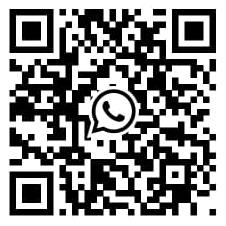# Two Sample test

The two-sample test is widely utilised to determine while two population means are similar. It is mostly used hypothesis tests in Six Sigma work. The application is used to test whether any of the new processes or treatments is better than a present treatment or process. It cross-checks whether the implementation of a new sales tool increases sales than before. This test is done when the two small samples (n< 30) are taken from two different populations and compared. By the use of the Two-Sample Test to perform if the means of two independent sets differ. This two-sample test calculates values possible to contain the distinction between the population means. The two-sample t-test is useful to calculate the hypothesis and confidence level of the difference among the population means while the standard deviation is unidentified as well as samples sketched free from each other.

## Assumption of Two Sample test includes:-

• A random selection of the sample is done from the two population
• Samples are independent of each other
• The count of sizes must be less than 30
• The samples collected are normally distributed

## Two variables are required to perform the two-sample test:-

• one variable defines the two groups
• and the second variable defines the measurement of interest

### There are two types of Two Sample tests!

1. Two Sample T Hypothesis Test (Equal Variance)
2. The variance of two populations are equal
3. Two Sample T Hypothesis Test (Unequal Variance)
4. The variance of two populations are NOT equal

### Two Sample T Hypothesis Test (Equal Variance) formula

1. Where n1 and n2 are sample sizes
2. x̅1 and x̅2 are means of sample sizes
3. Sp is the pooled standard deviation

### Two Sample T Hypothesis Test (Unequal Variance) formula

1. Where n1 and n2 are sample sizes
2. S12 & S22 are variances of sample 1 and sample 2
3. x̅1 and x̅2 are means of sample sizes

## Two-sample t-tests answer the following questions:-

1. Is process 1 equivalent to process 2?
2. Is the new process better than the current one?
3. There should be some pre-determined threshold amount between the new
4. process and the current process.

Over the past 10 years, the SPSS tutor has been known as being the expert in SPSS consulting and statistical operations. We dedicatedly work with our experts and ensure your model is robust—accounting for market efficiency and other determining factors—to maximize the probability of profit.

You can also send us an email   or call us directly on, (+44) 20 3287 0255 and will get in touch with you shortly

I had to submit a big report within a few days. I thought it was impossible to do so. But experts from SPSS Tutor Saved the day.

Paul

had complex research about a medical condition. It was difficult to finish the tasks. But thanks to the SPSS tutor, they helped me to finish tasks on time and made it look easy.

Emily

I had to write an assignment for an IT topic. It required research on new technology. Finding information about it was difficult because it is new. But the SPSS tutor team helped me with it and today I got the highest grades in the class.

Andrew

They helped me with my statistics homework. The team is friendly, the service is nice and I liked how they kept asking me suggestions. I loved their service and would recommend it to others.

Kimberly

Whatsapp Scan